﻿ 用卷积神经网络估计离散选择模型 Estimate Discrete Choice Models with Convolutional Neural Network – 王灿的个人网站

# 0.  概要 Outline

This article presents a method of estimating discrete choice models using convolutional neural network (CNN), so that the powerful predicting abilities of neural network could be utilized in choice situations. Three results are shown through experiments: 1) a MNL model is completely equivalent to a CNN with 1 convolutional layer, in which there only 1 out channel and no nonlinear activation; 2)  CNN with multiple convolutional layers + multiple out channels in each layer + nonlinear activation could greatly solve the nonlinear utility problem which is nearly inevitable in practice; 3)however, such CNN could not solve the problems concerning the more complex discrete choice models, such as nested logit model.

When a MNL model needs to be improved, there are generally 2 main directions. The one is to better specify the utilities, such as introducing nonlinear terms; the other is to replace the MNL form using more refined NL, MXL, etc. From the findings above, the former could be achieved by CNN, while the latter not.

There experiments are conducted using 3 pieces of Python codes in ‘PythonCode.zip’. ‘identical_simple_cnn_mnl.py’ shows a simple CNN could be equivalent to MNL; ‘complex_cnn.py’ shows how CNN well performs when utilities in MNL are nonlinear; ‘nested_logit.py’ shows CNN is incapable of more complex models, such as nested logit model.

PythonCode.zip

# 1.  用CNN实现MNL (Generating the Same Results of MNL Using CNN)

Neural network has outstanding reputation in many kinds of prediction problems, however, it is seldom used in predicting individual choices. At the same time, discrete choice models generally assume linear utilities, which are problematic in many occasions and thus need the powerful nonlinearity of neural network.

People may believe that we could generalize the applications of neural network in classification to choice, since the dependent variables are discrete in both classification and choice. However, it might not that easy, because the data format are different in classification and choice problems. For any explanatory variable,  one case usually has ONLY ONE value in a classification problem; by contrast, in a choice problem with N alternatives, one case usually has N dependent values for certain explanatory variable, that is to say, each alternative would have a corresponding value. For example, when travelers choose traffic modes among bus/ taxi /walk according travel time, each mode has its own travel time. Consequently, it is general to have only one row for a case in a classification problem, while in a N-alternative choice problem, a case often need N rows to be a group, as shown in the following table, 2 cases corresponds to 6 rows.

 Group Alt=alternative Choice TT = travel time (min) Price (￥) 1 Bus 1 20 2 1 Taxi 0 10 20 1 Walk 0 70 0 2 Bus 0 40 5 2 Taxo 0 10 20 2 Walk 1 100 0

How to deal with this kind of data using neural network? If we adopt classical fully connected neural network, 6 inputs nodes are needed to handle the 2 variables (TT and Price), which stand for ‘Bus TT’, ‘Taxi TT’, ‘Walk TT’, ‘Bus Price’, ‘Taxi Price’, ‘Walk Price’ respectively. This way should also work, but seems a little bit strange.

As we all know, a fully connected neural network without hidden layers and nonlinear activation functions would just equal to a linear regression.  Similarly, I wonder if there is a certain kind of neural network completely equal to MNL.

Let’s revisit the specification of MNL. In the case above, we could use two coefficients, b(TT) and b(Price) to specify a MNL model, as shown below. Constants are omitted for simplicity.

V(Bus) = b(TT) * TT(Bus)  +  b(Price) * Price(Bus)
V(Taxi) = b(TT) * TT(Taxi)  +  b(Price) * Price(Taxi)
V(Walk) = b(TT) * TT(Walk)  +  b(Price) * Price(Walk)

P(Bus) = exp(Bus)  / (exp(Bus)  +  exp(Taxi)  +  exp(Walk))
P(Taxi) = exp(Taxi)  / (exp(Bus)  +  exp(Taxi)  +  exp(Walk))
P(Walk) = exp(Walk)  / (exp(Bus)  +  exp(Taxi)  +  exp(Walk))

Such specifications remind me of convolution. What we are doing in calculating utilities (V) is actually a special kind of convolutional process by scanning each alternative using a fixed row vector [b(TT), b(Price)]. We could treat a N-row case as a picture, whose height is the number of alternatives (NALT), and whose length is the number of variables (NVAR), and this convolutional process is to scan each row of the picture using a ‘1 * NVAR’ row-kernel, and output a ‘NALT * 1’ column-picture. Finally, calculating probabilities according to utilities is a softmax process.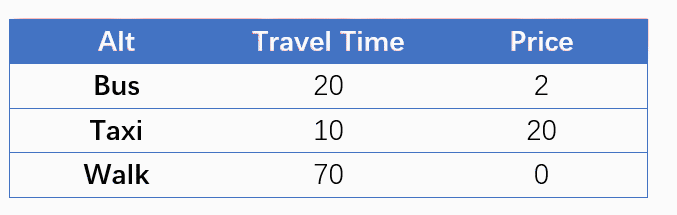Following this way, I tried to set up a MNL model using CNN. For a choice situation with NALT alternatives and NVAR explanatory variables, the tensorflow codes for CNN is as follows.

X = tf.placeholder(tf.float32, (None, NALT,  NVAR, 1))
Y = tf.placeholder(tf.float32, (None, NALT))
W = tf.get_variable(‘W’, [1,NVAR,1,1], initializer=tf.zeros_initializer())
Z = tf.nn.conv2d(X, W, (1,1,1,1),”VALID”)
Z2 = tf.contrib.layers.flatten(Z)
loss = tf.reduce_mean(tf.nn.softmax_cross_entropy_with_logits(labels=Y, logits=Z2))

The input dimension says that each case is a ‘picture’ with the size of ‘NALT * NVAR’ and one channel. The size of the convolutional weight ‘W’ is ‘1 * NVAR’, one input channel corresponds to the one channel of X, and one output channel means there is only one filter. There are no constants, no nonlinear activation, and no pooling layers, the whole calculation is to generate ‘Z’ using a linear convolution filter. From ‘Z’ to ‘Z2’ using flatten function is purely for the dimension, and the cross entropy loss is derived from ‘Z2’ and the observation ‘Y’. Finally, minimizing the cross entropy loss equals to maximizing the log likelihood.

Conducting experiments in the following way. First, randomly generate data and coefficients; second, simulate the choice results according the probabilities derived from the MNL model; third, treat the simulated choice results as dependent variables, establish a MNL model and a CNN model.

The following picture shows the estimating result of a random trial with 10 alternatives, 10 explanatory variables and 1000 choice situations. It could be seen that 1) the absolute values of the initial / final log likelihood and the initial / final cross entropy are exactly the same; 2) although CNN model is trained for 1000 steps, it converge actually in less than 200 steps and then just does nothing; 3) the coefficients of MNL and CNN are exactly the same.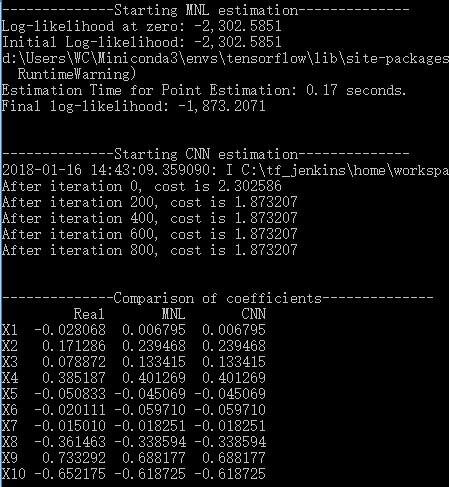Consequently, this experiment proves that a simple CNN could function exactly as MNL given certain specification. Moreover, each parameter of this CNN is explainable,  although in most other CNNs we do not care much about the explanation.

# 2.  非线性的解释变量：CNN的优势 (Nonlinear Utilities: Advantage of CNN)

Before go further, let’s take a small transformation to turn the above data-table sideways. All alternatives are still stacked on the hight dimension, however, the explanatory variables, which were stacked on the length dimension before, are now turned to the depth dimension, that means the new picture would have a size of ‘NALT * 1’, and NVAR channels, as shown in the figure below.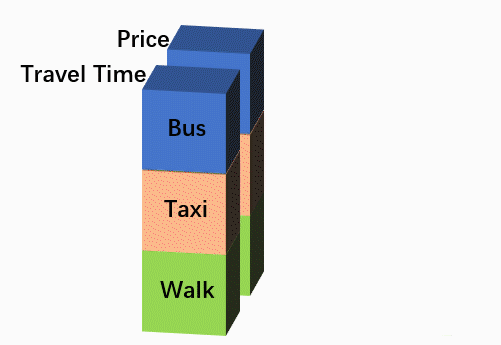Such transformation is to use multiple filters in a  layer more intuitively. The ‘Utility’ in the above figure is derived from a certain filter [b(TT), b(Pr)], while a typical CNN would use multiple filters. Each filter will generate a ‘NALT * 1’ output picture, which could be deemed as a new feature coming from the original features. Multiple output-features derived from multiple filters are then similarly stacked on the depth dimension. Supposing we have 4 filters, such calculations are the learning process from the original 2 features ‘Travel Time, Price’ to the new 4 features ‘Feature1, Feature2, Feature3, Feature4’. Moreover, a typical CNN may contains multiple convolutional layers, thus we could similarly generate ‘NextLayerFeature1, NextLayerFeature2, …, NextLayerFeatureN’ from ‘Feature1, Feature2, Feature3, Feature4’. This learning process just goes on, until in the last layer only one filter is used to generate the final score ‘Utility’, which is then sent to the softmax layer.The whole network structure is as follows.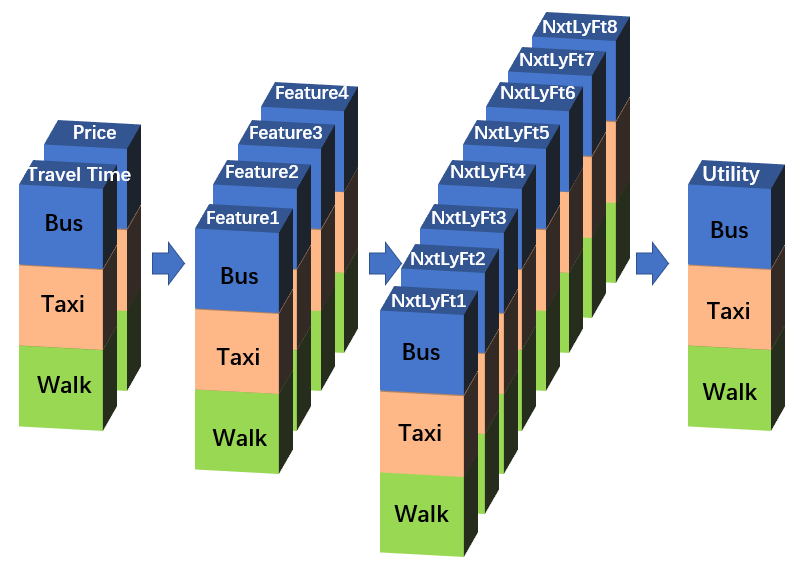Comparing with the simple CNN which is equivalent to MNL, this CNN further employs the advantages of neural networks in 3 aspects: 1) there are multiple filters in each convolutional layer; 2) there are multiple convolutional layers, thus a simple deep learning; 3) each convolutional layer is activated non-linearly.

However, this CNN also differs with typical CNNs for computer vision tasks in the following aspects: 1) the size of the sample ‘picture’ keeps ‘NALT * 1’, and the size of the filter keeps ‘1*1’; 2) no pooling layer; 3) no extra padding and stride considerations. Actually, this network just use the extrinsic form of CNN, and might be better described as ‘pseudo CNN’.

Now let’s check out the abilities of the ‘pseudo CNN’. One of the greatest problems of traditional discrete choice models is the linear specification of the utilities, which is unreliable in most cases. We could deliberately design a set of choice data with nonlinear utilities: suppose we observe 4 explanatory variables (X1, X2, X3, X4), and they are interacting with each other to constitute the utility, V = b1*X1*X2 + b2*X2*X3 + b3*X3*X4 + b4*X4*X1, the number of alternatives is 4, and the number of choice situations is 1000.

We estimate 3 models. MNL1 is the MNL model directly using X1~X4 as independent variables; MNL2 is the MNL model using Feature1~Feature4, where Feature1=X1*X2， Feature2=X2*X3，Feature3=X3*X4，Feature4=X4*X1, please notice that this model can not be estimate in practice, since we should have no idea about the real relationship between Feature1~Feature4 and X1~X4; the third one is the CNN model directly using X1~X4, this network contains 3 convolutional layers,  each has 20, 40, 1 filters, the first 2 layers have Relu activation and the last layer has no nonlinear activation. The whole data is split into training and test set (70%:30%). The estimating results are as follows.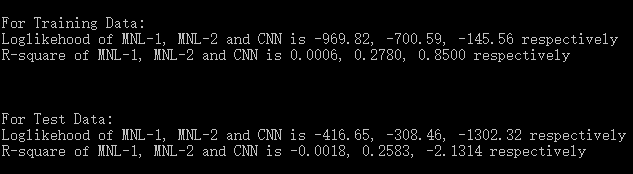MNL1 has a quite poor performance, indicating its inability to the nonlinearity. MNL2, the ‘true model’, has similar performance in both training and test data, showing robustness of MNL. In contrast, CNN has a unbelievable wonderful goodness of fit in the training data, and extraordinary poor performance in the test data, which fall far behind randomly guess.  Apparently there is a serious over-fitting in CNN. Nevertheless, its great performance in the training data shows the strong power of neural network.

There are many ways to deal with over-fitting in neural network, here we simply  add a L2 regularization and re-experiment.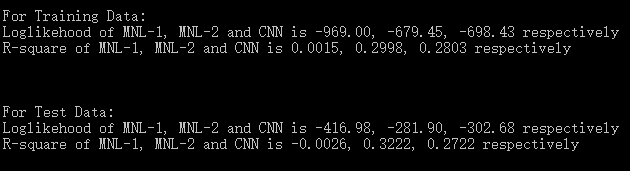For the new data, MNL1 / MNL2 keeps very bad / good  performance. The over-fitting problem of CNN is basically solved, it falls slightly behind MNL2  in both training and test data , but  greatly outperforms MNL1. Consequently, it is safe to say that when the utilities have nonlinear forms, CNN is superior to MNL.  On the other hand, MNL has its own advantages in simplicity and interpretability, while CNN is purely a predicting tool and prone to over-fitting.

I have also tried some other forms of nonlinear utilities, 3 of which are given in the codes. For every nonlinear utility form, CNN outperform MNL.

# 3.  复杂的选择模型形式：CNN无能为力 (More Complex Choice Model: Inability of CNN)

Furthermore, I expect if CNN could implement more complicated discrete choice models, such as nested logit (NL), mixed logit (MXL). Supposing we have a choice problem that should be treated using NL because of correlations among alternatives, but we still employ simple MNL and get an unsatisfactory result. Then how about CNN? Let’s try new experiments.

Assuming each two adjacent alternatives (Alt1 & Alt2, Alt3 & Alt4,…) are in the same nest, and each nest has a parameter lambda, representing the degree of correlation among the two alternatives in this nest. 3000 choice situations are randomly generated according to this specification, with 6 alternatives and 10 explanatory variables. 3 models, MNL, NL, and CNN are estimated. The CNN model has the same network structure as before and also use L2 regularization. The results are as follows.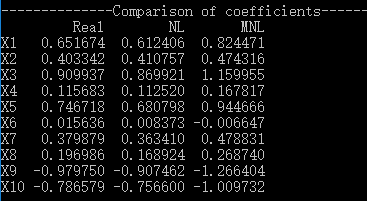First, let’s compare the true coefficients and estimated coefficients of MNL and NL. It is clear that NL coefficients are closer to the true settings. Although the parameters of different models should not be compared directly, the MNL results are apparently biased.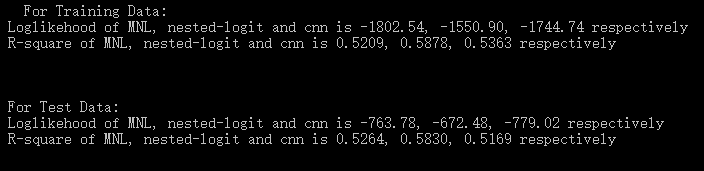As to goodness of fit, NL, the true model,  has the best performance unsurprisingly. Compared with MNL, CNN performs slightly better in the training data, while slightly worse in the test data. That is to say, CNN has no advantages over simple MNL, it could no handle the complex tree structure in the NL.

I would not spend time in more complicated MXL model, and just believe that these refined discrete choice models are irreplaceable by CNN.

### This Post Has 5 Comments

1.请问一下，第2节中出现的V = b1*X1*X2 + b2*X2*X3 + b3*X3*X4 + b4*X4*X1，可能会出现这种效用表达式吗？如果有，代表什么含义呢？谢谢

1.X1, X2, X3, X4的两两相乘只是一种抽象的算例，并没有实际意义。
不过，如果你问的是两个解释变量相乘有没有意义，是有的，代表交互效应或调节效应。一个简单的例子是：X1代表性别（0-男，1-女），X2代表价格，那么b1*X1*X2这一项中的b1就反映了男女在价格敏感度上的差异

2.有没有可能后面cnn效果不好的原因，就是因为只用了1×1的卷积核。如果把他适当扩大，也许能学到哪些选项之间存在关系。虽然不会像NL那样有显式的预设公式，但是可以学出unobservable的相关性。

3.补充一下，上面说的相关性是备选项之间的相似性

4.我可能应该收回上面的问题，不过你最后还是需要得到NALT个Utility，所以从目前看1×1似乎不能避免。

ICP备案号：沪ICP备20010289号-1